This book is archived and will be removed July 6, 2022. Please use the updated version.

Photons and Matter Waves

# 44 Photoelectric Effect

### Learning Objectives

By the end of this section you will be able to:

• Describe physical characteristics of the photoelectric effect
• Explain why the photoelectric effect cannot be explained by classical physics
• Describe how Einstein’s idea of a particle of radiation explains the photoelectric effect

When a metal surface is exposed to a monochromatic electromagnetic wave of sufficiently short wavelength (or equivalently, above a threshold frequency), the incident radiation is absorbed and the exposed surface emits electrons. This phenomenon is known as the photoelectric effect. Electrons that are emitted in this process are called photoelectrons.

The experimental setup to study the photoelectric effect is shown schematically in (Figure). The target material serves as the anode, which becomes the emitter of photoelectrons when it is illuminated by monochromatic radiation. We call this electrode the photoelectrode. Photoelectrons are collected at the cathode, which is kept at a lower potential with respect to the anode. The potential difference between the electrodes can be increased or decreased, or its polarity can be reversed. The electrodes are enclosed in an evacuated glass tube so that photoelectrons do not lose their kinetic energy on collisions with air molecules in the space between electrodes.

When the target material is not exposed to radiation, no current is registered in this circuit because the circuit is broken (note, there is a gap between the electrodes). But when the target material is connected to the negative terminal of a battery and exposed to radiation, a current is registered in this circuit; this current is called the photocurrent. Suppose that we now reverse the potential difference between the electrodes so that the target material now connects with the positive terminal of a battery, and then we slowly increase the voltage. The photocurrent gradually dies out and eventually stops flowing completely at some value of this reversed voltage. The potential difference at which the photocurrent stops flowing is called the stopping potential.

An experimental setup to study the photoelectric effect. The anode and cathode are enclosed in an evacuated glass tube. The voltmeter measures the electric potential difference between the electrodes, and the ammeter measures the photocurrent. The incident radiation is monochromatic.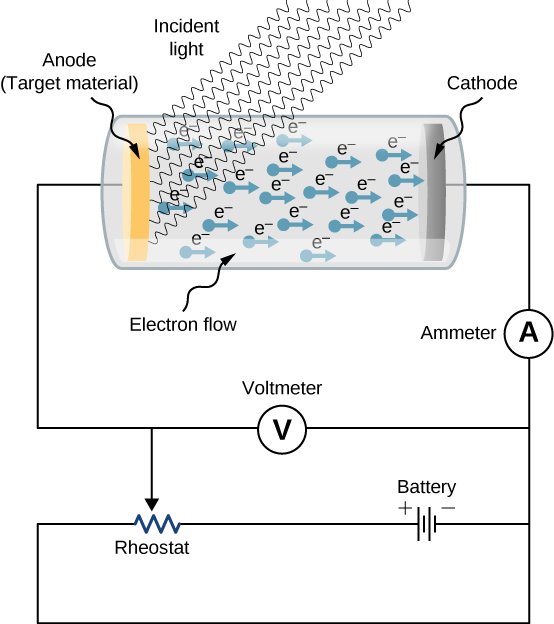### Characteristics of the Photoelectric Effect

The photoelectric effect has three important characteristics that cannot be explained by classical physics: (1) the absence of a lag time, (2) the independence of the kinetic energy of photoelectrons on the intensity of incident radiation, and (3) the presence of a cut-off frequency. Let’s examine each of these characteristics.

#### The absence of lag time

When radiation strikes the target material in the electrode, electrons are emitted almost instantaneously, even at very low intensities of incident radiation. This absence of lag time contradicts our understanding based on classical physics. Classical physics predicts that for low-energy radiation, it would take significant time before irradiated electrons could gain sufficient energy to leave the electrode surface; however, such an energy buildup is not observed.

#### The intensity of incident radiation and the kinetic energy of photoelectrons

Typical experimental curves are shown in (Figure), in which the photocurrent is plotted versus the applied potential difference between the electrodes. For the positive potential difference, the current steadily grows until it reaches a plateau. Furthering the potential increase beyond this point does not increase the photocurrent at all. A higher intensity of radiation produces a higher value of photocurrent. For the negative potential difference, as the absolute value of the potential difference increases, the value of the photocurrent decreases and becomes zero at the stopping potential. For any intensity of incident radiation, whether the intensity is high or low, the value of the stopping potential always stays at one value.

To understand why this result is unusual from the point of view of classical physics, we first have to analyze the energy of photoelectrons. A photoelectron that leaves the surface has kinetic energy K. It gained this energy from the incident electromagnetic wave. In the space between the electrodes, a photoelectron moves in the electric potential and its energy changes by the amount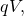where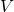is the potential difference and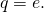Because no forces are present but electric force, by applying the work-energy theorem, we obtain the energy balance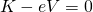for the photoelectron, where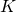is the change in the photoelectron’s kinetic energy. When the stopping potential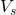is applied, the photoelectron loses its initial kinetic energy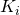and comes to rest. Thus, its energy balance becomes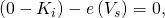so that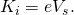In the presence of the stopping potential, the largest kinetic energy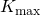that a photoelectron can have is its initial kinetic energy, which it has at the surface of the photoelectrode. Therefore, the largest kinetic energy of photoelectrons can be directly measured by measuring the stopping potential: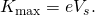At this point we can see where the classical theory is at odds with the experimental results. In classical theory, the photoelectron absorbs electromagnetic energy in a continuous way; this means that when the incident radiation has a high intensity, the kinetic energy in (Figure) is expected to be high. Similarly, when the radiation has a low intensity, the kinetic energy is expected to be low. But the experiment shows that the maximum kinetic energy of photoelectrons is independent of the light intensity.

The detected photocurrent plotted versus the applied potential difference shows that for any intensity of incident radiation, whether the intensity is high (upper curve) or low (lower curve), the value of the stopping potential is always the same.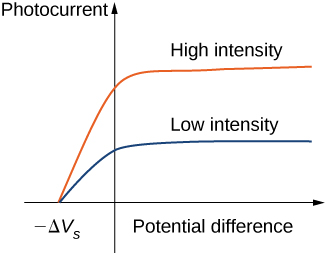#### The presence of a cut-off frequency

For any metal surface, there is a minimum frequency of incident radiation below which photocurrent does not occur. The value of this cut-off frequency for the photoelectric effect is a physical property of the metal: Different materials have different values of cut-off frequency. Experimental data show a typical linear trend (see (Figure)). The kinetic energy of photoelectrons at the surface grows linearly with the increasing frequency of incident radiation. Measurements for all metal surfaces give linear plots with one slope. None of these observed phenomena is in accord with the classical understanding of nature. According to the classical description, the kinetic energy of photoelectrons should not depend on the frequency of incident radiation at all, and there should be no cut-off frequency. Instead, in the classical picture, electrons receive energy from the incident electromagnetic wave in a continuous way, and the amount of energy they receive depends only on the intensity of the incident light and nothing else. So in the classical understanding, as long as the light is shining, the photoelectric effect is expected to continue.

Kinetic energy of photoelectrons at the surface versus the frequency of incident radiation. The photoelectric effect can only occur above the cut-off frequency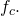Measurements for all metal surfaces give linear plots with one slope. Each metal surface has its own cut-off frequency.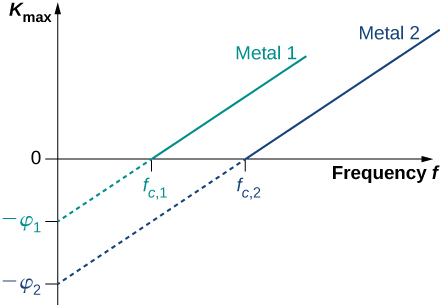### The Work Function

The photoelectric effect was explained in 1905 by A. Einstein. Einstein reasoned that if Planck’s hypothesis about energy quanta was correct for describing the energy exchange between electromagnetic radiation and cavity walls, it should also work to describe energy absorption from electromagnetic radiation by the surface of a photoelectrode. He postulated that an electromagnetic wave carries its energy in discrete packets. Einstein’s postulate goes beyond Planck’s hypothesis because it states that the light itself consists of energy quanta. In other words, it states that electromagnetic waves are quantized.

In Einstein’s approach, a beam of monochromatic light of frequency f is made of photons. A photon is a particle of light. Each photon moves at the speed of light and carries an energy quantum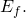A photon’s energy depends only on its frequency f. Explicitly, the energy of a photon is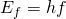where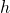is Planck’s constant. In the photoelectric effect, photons arrive at the metal surface and each photon gives away all of its energy to only one electron on the metal surface. This transfer of energy from photon to electron is of the “all or nothing” type, and there are no fractional transfers in which a photon would lose only part of its energy and survive. The essence of a quantum phenomenon is either a photon transfers its entire energy and ceases to exist or there is no transfer at all. This is in contrast with the classical picture, where fractional energy transfers are permitted. Having this quantum understanding, the energy balance for an electron on the surface that receives the energy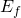from a photon is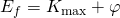whereis the kinetic energy, given by (Figure), that an electron has at the very instant it gets detached from the surface. In this energy balance equation,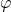is the energy needed to detach a photoelectron from the surface. This energyis called the work function of the metal. Each metal has its characteristic work function, as illustrated in (Figure). To obtain the kinetic energy of photoelectrons at the surface, we simply invert the energy balance equation and use (Figure) to express the energy of the absorbed photon. This gives us the expression for the kinetic energy of photoelectrons, which explicitly depends on the frequency of incident radiation: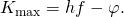This equation has a simple mathematical form but its physics is profound. We can now elaborate on the physical meaning behind (Figure).

Typical Values of the Work Function for Some Common Metals
Metal(eV)
Na 2.46
Al 4.08
Pb 4.14
Zn 4.31
Fe 4.50
Cu 4.70
Ag 4.73
Pt 6.35

In Einstein’s interpretation, interactions take place between individual electrons and individual photons. The absence of a lag time means that these one-on-one interactions occur instantaneously. This interaction time cannot be increased by lowering the light intensity. The light intensity corresponds to the number of photons arriving at the metal surface per unit time. Even at very low light intensities, the photoelectric effect still occurs because the interaction is between one electron and one photon. As long as there is at least one photon with enough energy to transfer it to a bound electron, a photoelectron will appear on the surface of the photoelectrode.

The existence of the cut-off frequency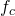for the photoelectric effect follows from (Figure) because the kinetic energyof the photoelectron can take only positive values. This means that there must be some threshold frequency for which the kinetic energy is zero,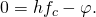In this way, we obtain the explicit formula for cut-off frequency: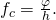Cut-off frequency depends only on the work function of the metal and is in direct proportion to it. When the work function is large (when electrons are bound fast to the metal surface), the energy of the threshold photon must be large to produce a photoelectron, and then the corresponding threshold frequency is large. Photons with frequencies larger than the threshold frequencyalways produce photoelectrons because they have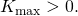Photons with frequencies smaller thando not have enough energy to produce photoelectrons. Therefore, when incident radiation has a frequency below the cut-off frequency, the photoelectric effect is not observed. Because frequency f and wavelength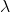of electromagnetic waves are related by the fundamental relation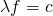(where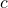is the speed of light in vacuum), the cut-off frequency has its corresponding cut-off wavelength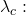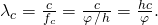In this equation,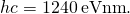Our observations can be restated in the following equivalent way: When the incident radiation has wavelengths longer than the cut-off wavelength, the photoelectric effect does not occur.

Photoelectric Effect for Silver Radiation with wavelength 300 nm is incident on a silver surface. Will photoelectrons be observed?

Strategy Photoelectrons can be ejected from the metal surface only when the incident radiation has a shorter wavelength than the cut-off wavelength. The work function of silver is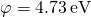((Figure)). To make the estimate, we use (Figure).

Solution The threshold wavelength for observing the photoelectric effect in silver is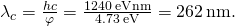The incident radiation has wavelength 300 nm, which is longer than the cut-off wavelength; therefore, photoelectrons are not observed.

Significance If the photoelectrode were made of sodium instead of silver, the cut-off wavelength would be 504 nm and photoelectrons would be observed.

(Figure) in Einstein’s model tells us that the maximum kinetic energy of photoelectrons is a linear function of the frequency of incident radiation, which is illustrated in (Figure). For any metal, the slope of this plot has a value of Planck’s constant. The intercept with the-axis gives us a value of the work function that is characteristic for the metal. On the other hand,can be directly measured in the experiment by measuring the value of the stopping potential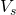(see (Figure)) at which the photocurrent stops. These direct measurements allow us to determine experimentally the value of Planck’s constant, as well as work functions of materials.

Einstein’s model also gives a straightforward explanation for the photocurrent values shown in (Figure). For example, doubling the intensity of radiation translates to doubling the number of photons that strike the surface per unit time. The larger the number of photons, the larger is the number of photoelectrons, which leads to a larger photocurrent in the circuit. This is how radiation intensity affects the photocurrent. The photocurrent must reach a plateau at some value of potential difference because, in unit time, the number of photoelectrons is equal to the number of incident photons and the number of incident photons does not depend on the applied potential difference at all, but only on the intensity of incident radiation. The stopping potential does not change with the radiation intensity because the kinetic energy of photoelectrons (see (Figure)) does not depend on the radiation intensity.

Work Function and Cut-Off Frequency When a 180-nm light is used in an experiment with an unknown metal, the measured photocurrent drops to zero at potential – 0.80 V. Determine the work function of the metal and its cut-off frequency for the photoelectric effect.

Strategy To find the cut-off frequency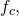we use (Figure), but first we must find the work function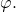To find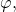we use (Figure) and (Figure). Photocurrent drops to zero at the stopping value of potential, so we identify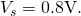Solution We use (Figure) to find the kinetic energy of the photoelectrons: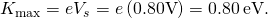Now we solve (Figure) for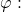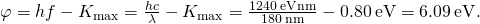Finally, we use (Figure) to find the cut-off frequency: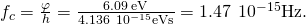Significance In calculations like the one shown in this example, it is convenient to use Planck’s constant in the units of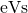and express all energies in eV instead of joules.

The Photon Energy and Kinetic Energy of Photoelectrons A 430-nm violet light is incident on a calcium photoelectrode with a work function of 2.71 eV.

Find the energy of the incident photons and the maximum kinetic energy of ejected electrons.

Strategy The energy of the incident photon is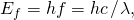where we use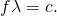To obtain the maximum energy of the ejected electrons, we use (Figure).

Solution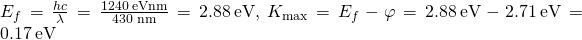Significance In this experimental setup, photoelectrons stop flowing at the stopping potential of 0.17 V.

Check Your Understanding A yellow 589-nm light is incident on a surface whose work function is 1.20 eV. What is the stopping potential? What is the cut-off wavelength?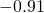V; 1040 nm

Check Your Understanding Cut-off frequency for the photoelectric effect in some materials is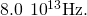When the incident light has a frequency of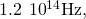the stopping potential is measured as – 0.16 V. Estimate a value of Planck’s constant from these data (in units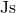and) and determine the percentage error of your estimation.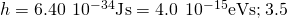### Summary

• The photoelectric effect occurs when photoelectrons are ejected from a metal surface in response to monochromatic radiation incident on the surface. It has three characteristics: (1) it is instantaneous, (2) it occurs only when the radiation is above a cut-off frequency, and (3) kinetic energies of photoelectrons at the surface do not depend of the intensity of radiation. The photoelectric effect cannot be explained by classical theory.
• We can explain the photoelectric effect by assuming that radiation consists of photons (particles of light). Each photon carries a quantum of energy. The energy of a photon depends only on its frequency, which is the frequency of the radiation. At the surface, the entire energy of a photon is transferred to one photoelectron.
• The maximum kinetic energy of a photoelectron at the metal surface is the difference between the energy of the incident photon and the work function of the metal. The work function is the binding energy of electrons to the metal surface. Each metal has its own characteristic work function.

### Conceptual Questions

For the same monochromatic light source, would the photoelectric effect occur for all metals?

No

In the interpretation of the photoelectric effect, how is it known that an electron does not absorb more than one photon?

Explain how you can determine the work function from a plot of the stopping potential versus the frequency of the incident radiation in a photoelectric effect experiment. Can you determine the value of Planck’s constant from this plot?

from the slope

Suppose that in the photoelectric-effect experiment we make a plot of the detected current versus the applied potential difference. What information do we obtain from such a plot? Can we determine from it the value of Planck’s constant? Can we determine the work function of the metal?

Speculate how increasing the temperature of a photoelectrode affects the outcomes of the photoelectric effect experiment.

Which aspects of the photoelectric effect cannot be explained by classical physics?

Is the photoelectric effect a consequence of the wave character of radiation or is it a consequence of the particle character of radiation? Explain briefly.

the particle character

The metals sodium, iron, and molybdenum have work functions 2.5 eV, 3.9 eV, and 4.2 eV, respectively. Which of these metals will emit photoelectrons when illuminated with 400 nm light?

### Problems

A photon has energy 20 keV. What are its frequency and wavelength?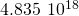Hz; 0.620 Å

The wavelengths of visible light range from approximately 400 to 750 nm. What is the corresponding range of photon energies for visible light?

What is the longest wavelength of radiation that can eject a photoelectron from silver? Is it in the visible range?

263 nm; no

What is the longest wavelength of radiation that can eject a photoelectron from potassium, given the work function of potassium 2.24 eV? Is it in the visible range?

Estimate the binding energy of electrons in magnesium, given that the wavelength of 337 nm is the longest wavelength that a photon may have to eject a photoelectron from magnesium photoelectrode.

3.68 eV

The work function for potassium is 2.26 eV. What is the cutoff frequency when this metal is used as photoelectrode? What is the stopping potential when for the emitted electrons when this photoelectrode is exposed to radiation of frequency 1200 THz?

Estimate the work function of aluminum, given that the wavelength of 304 nm is the longest wavelength that a photon may have to eject a photoelectron from aluminum photoelectrode.

4.09 eV

What is the maximum kinetic energy of photoelectrons ejected from sodium by the incident radiation of wavelength 450 nm?

A 120-nm UV radiation illuminates a gold-plated electrode. What is the maximum kinetic energy of the ejected photoelectrons?

5.54 eV

A 400-nm violet light ejects photoelectrons with a maximum kinetic energy of 0.860 eV from sodium photoelectrode. What is the work function of sodium?

A 600-nm light falls on a photoelectric surface and electrons with the maximum kinetic energy of 0.17 eV are emitted. Determine (a) the work function and (b) the cutoff frequency of the surface. (c) What is the stopping potential when the surface is illuminated with light of wavelength 400 nm?

a. 1.89 eV; b. 459 THz; c. 1.21 V

The cutoff wavelength for the emission of photoelectrons from a particular surface is 500 nm. Find the maximum kinetic energy of the ejected photoelectrons when the surface is illuminated with light of wavelength 600 nm.

Find the wavelength of radiation that can eject 2.00-eV electrons from calcium electrode. The work function for calcium is 2.71 eV. In what range is this radiation?

264 nm; UV

Find the wavelength of radiation that can eject 0.10-eV electrons from potassium electrode. The work function for potassium is 2.24 eV. In what range is this radiation?

Find the maximum velocity of photoelectrons ejected by an 80-nm radiation, if the work function of photoelectrode is 4.73 eV.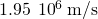### Glossary

cut-off frequency
frequency of incident light below which the photoelectric effect does not occur
cut-off wavelength
wavelength of incident light that corresponds to cut-off frequency
energy of a photon
quantum of radiant energy, depends only on a photon’s frequency
photocurrent
in a circuit, current that flows when a photoelectrode is illuminated
photoelectric effect
emission of electrons from a metal surface exposed to electromagnetic radiation of the proper frequency
photoelectrode
in a circuit, an electrode that emits photoelectrons
photoelectron
electron emitted from a metal surface in the presence of incident radiation
photon
particle of light
quantum phenomenon
in interaction with matter, photon transfers either all its energy or nothing
stopping potential
in a circuit, potential difference that stops photocurrent
work function
energy needed to detach photoelectron from the metal surface